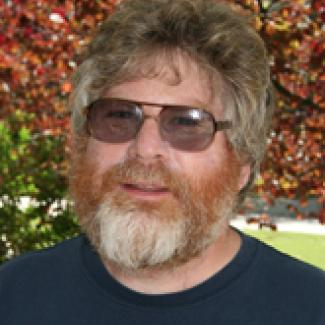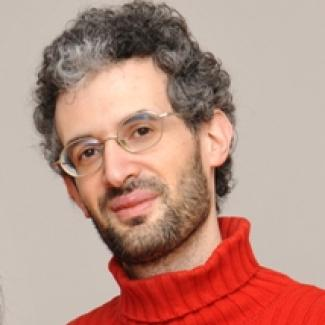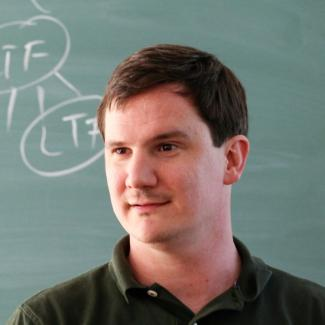Complexity theory, through such concepts as NP-completeness, distinguishes between computational problems that have relatively efficient solutions and those that are intractable. "Fine-grained" complexity aims to refine this qualitative distinction into a quantitative guide as to the exact time required to solve problems. This characterization makes sense for problems within P, distinguishing between, say, problems that require cubic time and those solvable in quadratic time. It may also guide us as to the exact complexity of hard problems, distinguishing, say, between NP-complete problems where exhaustive search is essentially the best possible algorithm, and those that have improved exponential time algorithms. Additionally, fine-grained complexity aims to precisely delineate the difficulty of instances of computational problems, finding parameters of instances that determine how much time algorithms will take on those instances. Such a complexity theory has the potential to become a real guide for algorithm design, identifying precisely what algorithmic performance is obtainable. In addition, fine-grained complexity is intimately linked to progress on traditional issues in computational complexity: in particular, it is essential to recent breakthroughs in circuit lower bounds via new algorithms, and is tightly linked to the question of derandomization of randomized algorithms.

This agenda, while broad and ambitious, is actually within reach! Recent research has made rapid progress on all of these fronts, and in fact the exciting possibilities mentioned above have been proved to be linked together. All of them emerge from studying the exact and parameterized complexities of NP-complete problems. The precise time complexity of Satisfiability and other NP-complete problems is now linked to progress on a variety of fundamental questions in the theory of computation, many in surprising and counter-intuitive ways. These connections include: the exact complexity of basic problems within P such as matrix multiplication, triangle detection and k-Sum; time-size trade-offs for data structures; circuit lower bounds; derandomization; and parameterized algorithms and complexity. Recent research keeps adding to this list, suggesting that there are even more connections yet to be discovered.

We propose to gather researchers from the computational complexity, algorithm design, parameterized algorithms and Sat-heuristic communities to strengthen and utilize these connections between complexity and algorithm design. Our goals are to develop new algorithms, to prove new lower bounds, to understand the exact time complexity of problems both rigorously and quantitatively, and to strengthen and exploit the connections between algorithm design and lower bounds. As a side effect, we expect to see new algorithms for basic problems (often utilizing techniques from lower bounds), as well as new circuit lower bounds (utilizing improved algorithms).

## OrganizersMohan Paturi (UC San Diego; chair)Russell Impagliazzo (UC San Diego)Dániel Marx (Hungarian Academy of Sciences)Virginia Vassilevska Williams (Massachusetts Institute of Technology)

## Research Fellows

Holger Dell (Saarland University and Cluster of Excellence, MMCI)